# Mean Value theorems, Intermediate first year Mathematics problems with solutions

Mathematics intermediate first year 1A and 1B solutions for some problems. These solutions are very simple to understand.

Junior inter 1A : Functions, mathematical induction, addition of vectors, trigonometric ratios upto transformations, trigonometric equations, hyperbolic functions, inverse trigonometric functions and properties of triangles.

Junior inter 1B : Locus, transformation of axes and three dimensional coordinates, straight lines, pair of straight lines, directions cosines & ratios, the planes and applications of derivatives.

Textbook exercise solutions links are also given.

You can see the solutions for junior inter maths 1b

1. Locus

The straight lines sa

Straight lines la

12. Rate measure

You can also see the solutions for junior inter maths 1A

3. Matrices

You can see the solutions for text book Maths 1A

Functions

Exercise 1(a)

Exercise 1(b)

Exercise 1(c)

Mathematical Induction

Exercise 2(a)

Matrices

Exercise 3(a)

Exercise 3(b)

Exercise 3(c)

Exercise 3(d)

Exercise 3(e)

Exercise 3(f)

Exercise 3(g)

Exercise 3(h)

Exercise 3(i)

Exercise 4(a)

Exercise 4(b)

Product of vectors

Exercise 5(a)

Exercise 5(b)

Exercise 5(c)

Trigonometric Ratios up to Transformations

Exercise 6(a)

Exercise 6(b)

Exercise 6(c)

Exercise 6(d)

Exercise 6(e)

Exercise 6(f)

Trigonometric Equations

Exercise 7(a)

Inverse Trigonometric Equations

Exercise 8(a)

Hyperbolic Functions

Exercise 9(a)

Properties of Triangles

Exercise 10(a)

Exercise 10(b)

Maths 1B solutions for

Locus

Exercise 1(a)

Transformation of Axes

Exercise 2(a)

The Straight Line

Three Dimensional Coordinates

Exercise 5(a)

Exercise 5(b)

Direction Cosines and Direction Ratios

Exercise 6(a)

Exercise 6(b)

The Plane

Exercise 7(a)

Limits and Continuity

Exercise 8(a)

Exercise 8(b)

Exercise 8(c)

Exercise 8(d)

Exercise 8(e)

Applications of Derivaties

## Mean value theorems solutions, Inter maths 1b.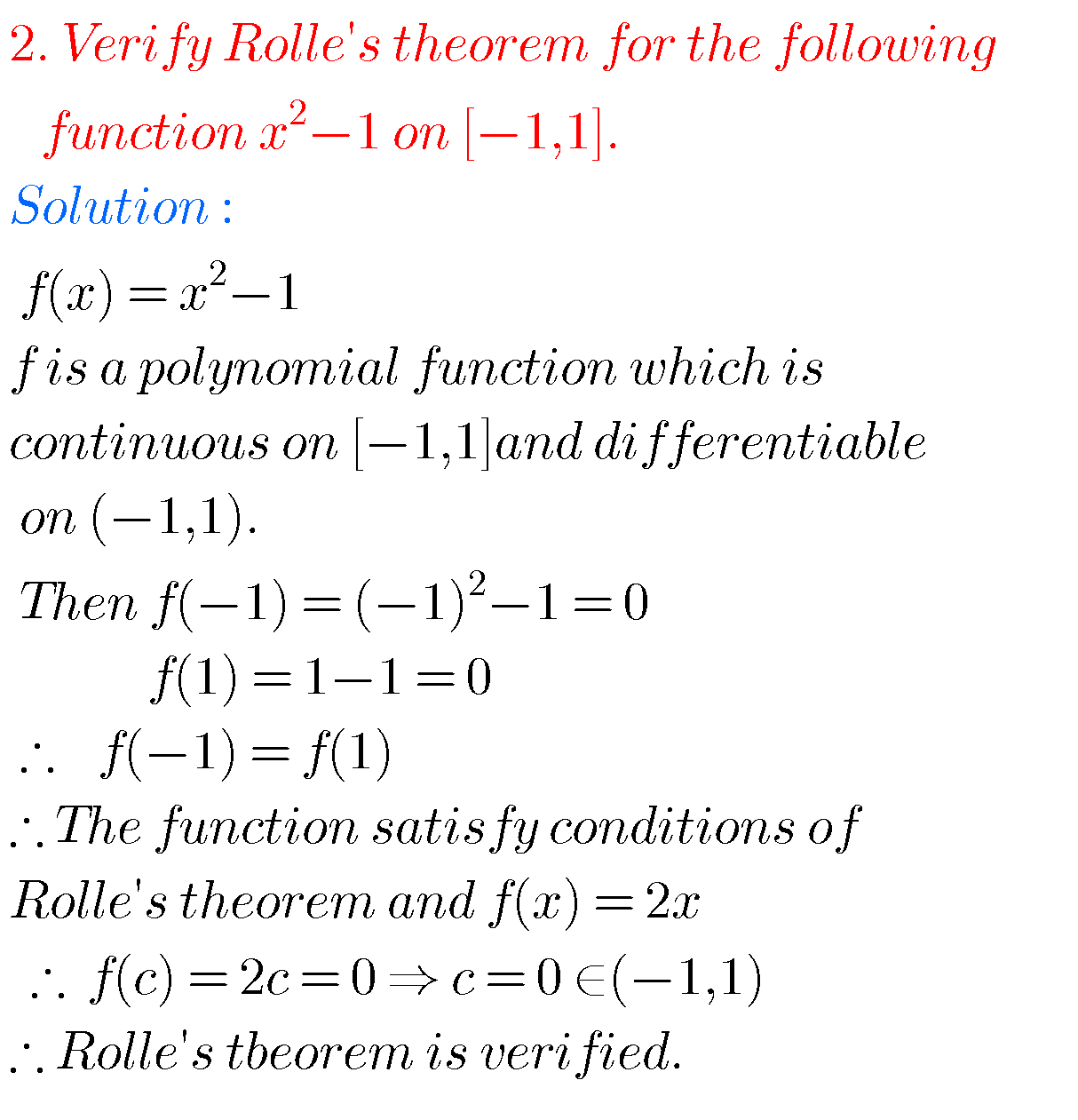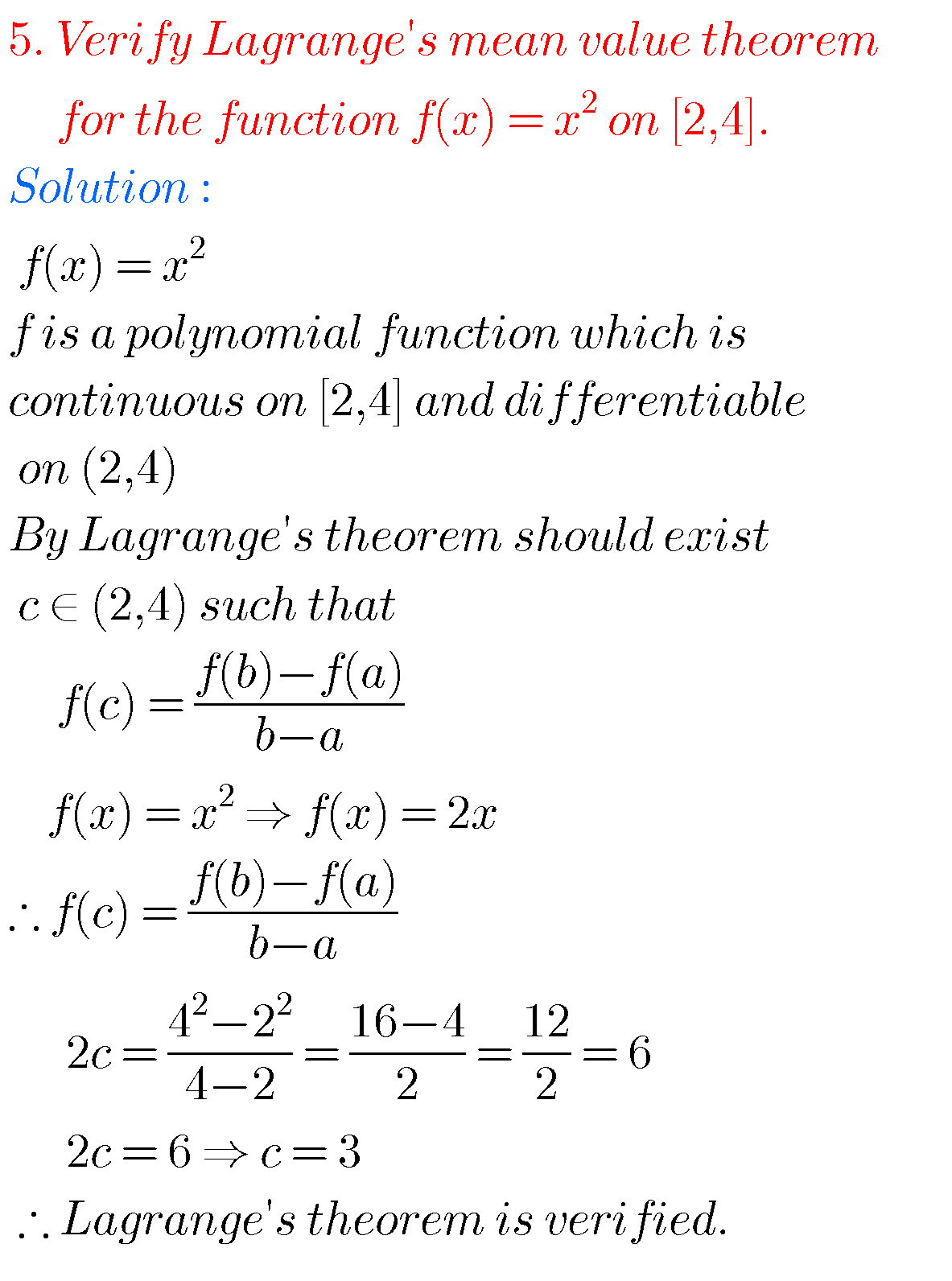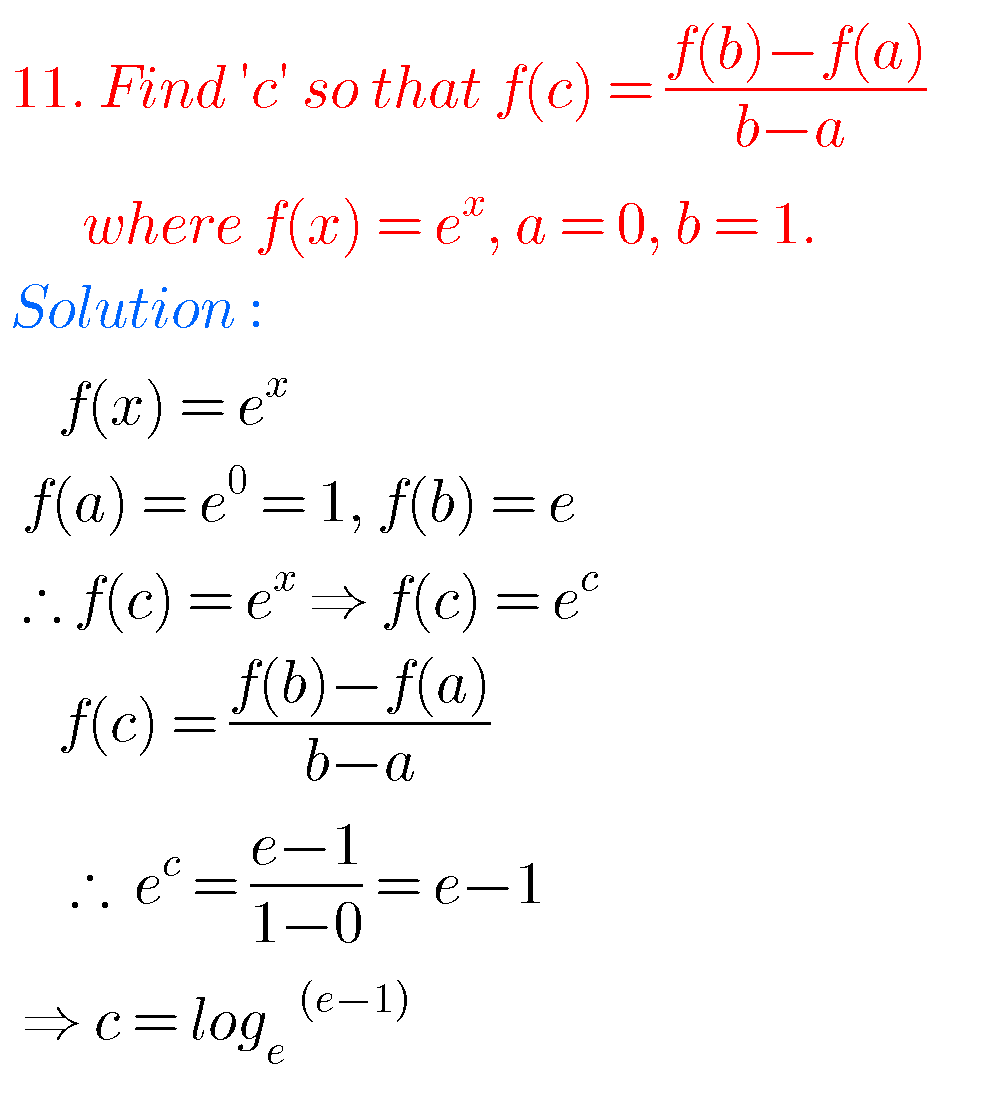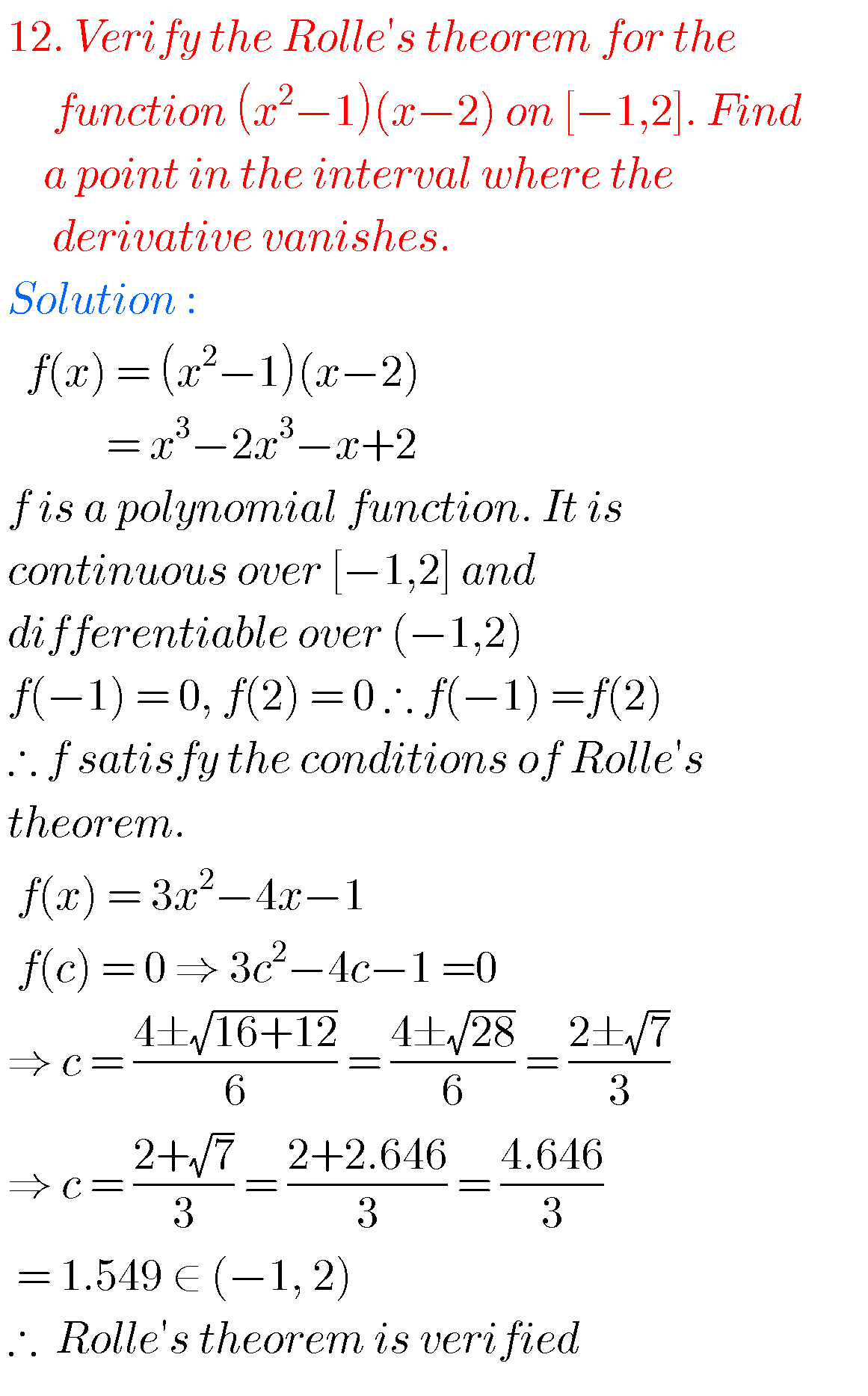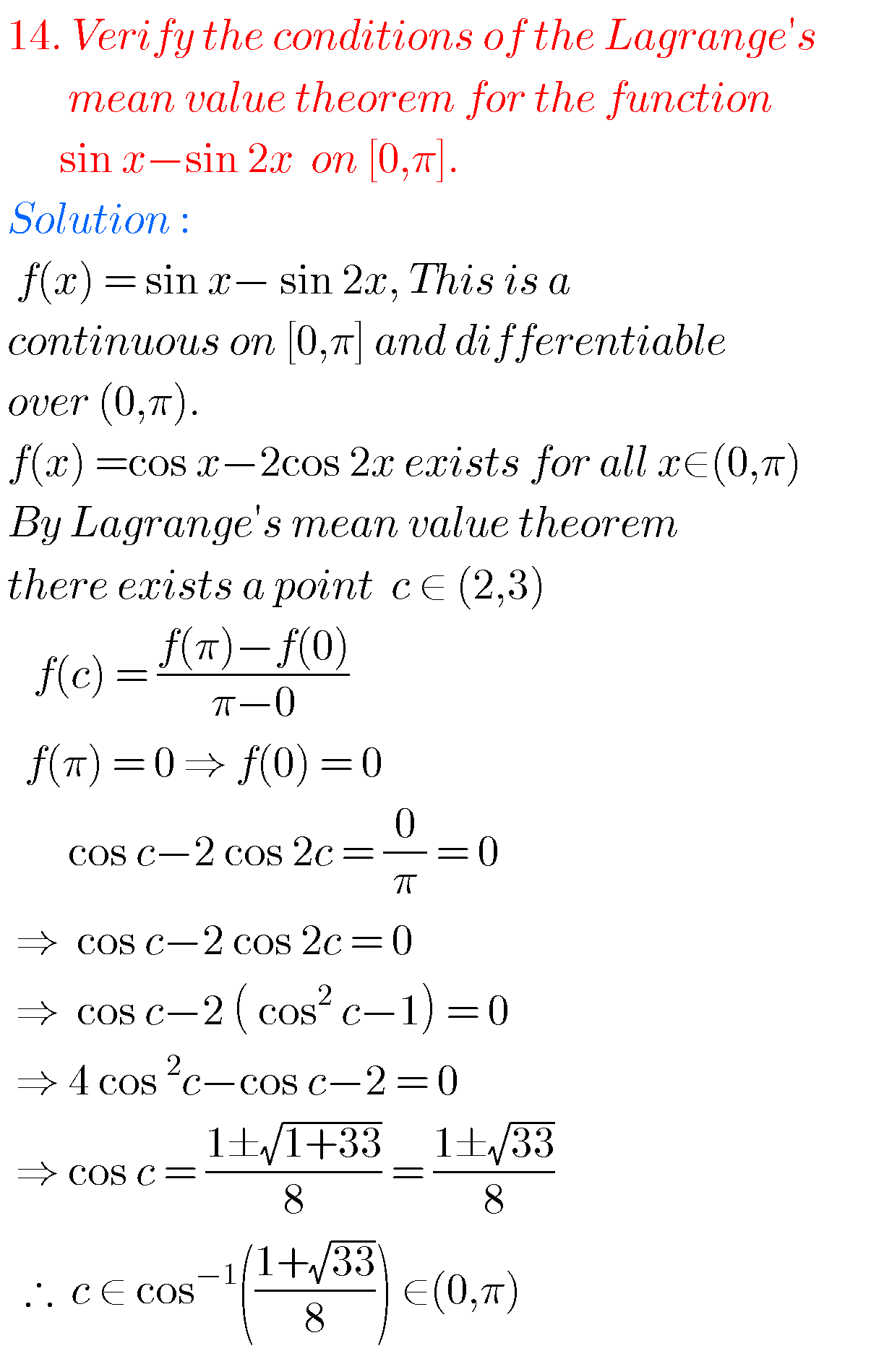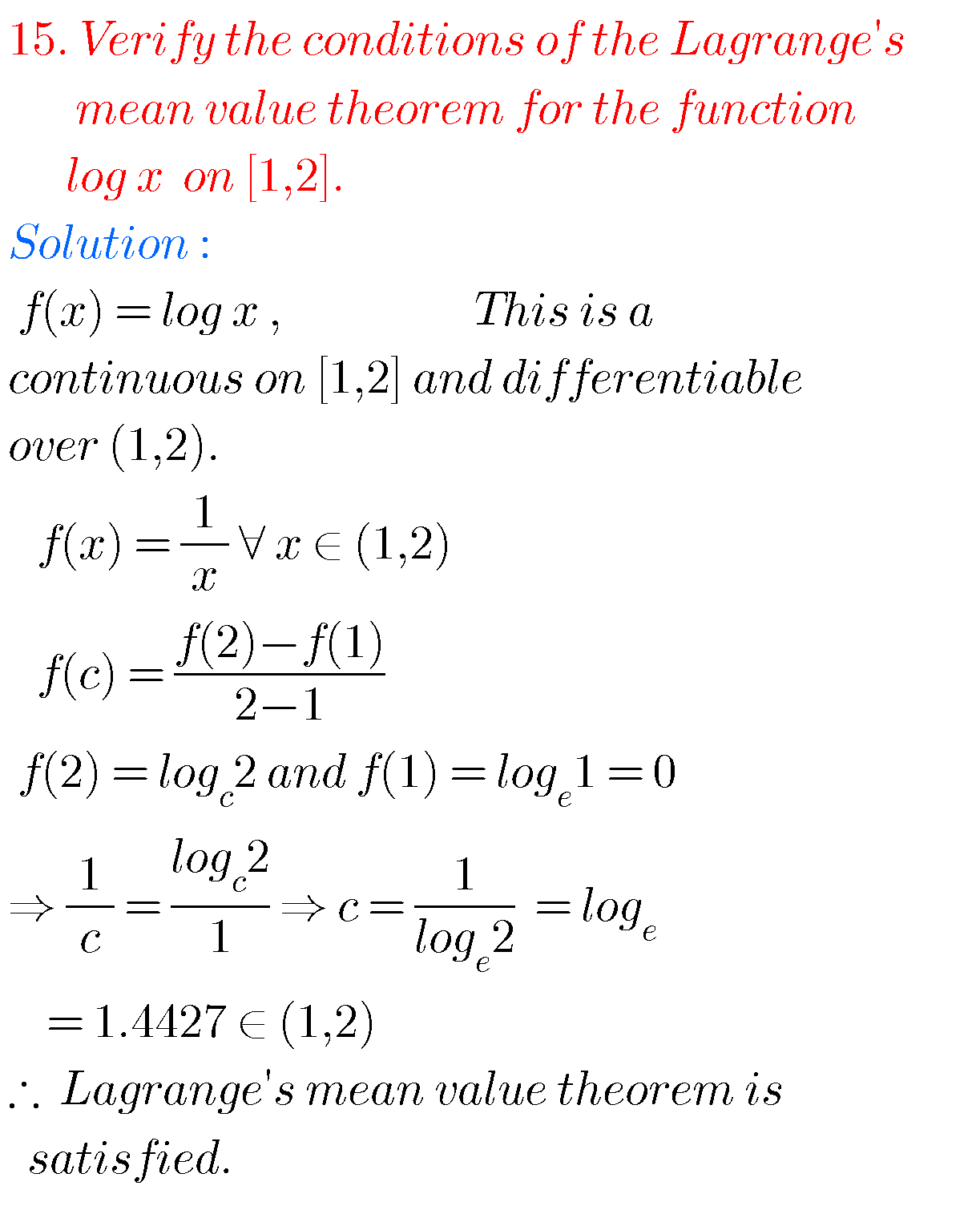Note : Observe the solutions and try them in your own methods.

Intermediate 1a solutions

Intermediate trigonometry solutions

Maths real numbers solutions class 10

You can see solutions for Inter Maths IIB

1. Circle

3. Parabola

4. Ellipse

You can also see solutions for Inter Maths IIA

For examination purpose you can see

Complex numbers

De Moivre’ s Theorem